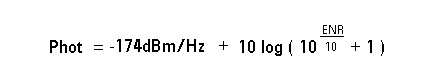Here’s the page we think you wanted. See search results instead:

Contact an Expert

# What is the absolute output power level of the various Keysight Technologies' noise sources?

The 346A/B/C family of noise source is controlled by +28 Vdc bias that turns the noise source on and off.

The off power is expressed by kTB.

Where:

• k is Boltzmann's constant (1.38E-23 joules/K)
• T is the temperature in K (Kelvin)
• B is the system's noise bandwidth

For T = 290 K, Pcold, then kTB is calculated as follows:

Pcold = kTB
Pcold = (1.38E-23 joules/K)*(290 K)/ 1 Hz
Pcold = (4.002E-21 watts/Hz)

Converting to dBW
Pcold = 10 * log10(4.002 E-21)/Hz
Pcold = -203.97 dBW/Hz

Converting dBW to dBm (1 Watt = + 30 dBm)
Pcold = (-203.97 dBW + 30 dB)/Hz
Pcold = -174 dBm/Hz

The Pcold changes by temperature, but the Phot is always constant.
The on power is calculated by ENR value, using the following equation:For example:
If the ENR value is 15 dB, then
Phot = -174 dBm/Hz + 10 * log(101.5 + 1)
Phot = -174 dBm/Hz + 10 * log(32.6)
Phot = -174 dBm/Hz + 15.1 dB
Phot = -158.9 dBm/Hz

And, if the ENR value is 5 dB, then
Phot = -174 dBm/Hz + 10 * log(100.5 + 1)
Phot = -174 dBm/Hz + 10 * log(4.16)
Phot = -174 dBm/Hz + 6.2 dB
Phot = -167.8 dBm/Hz